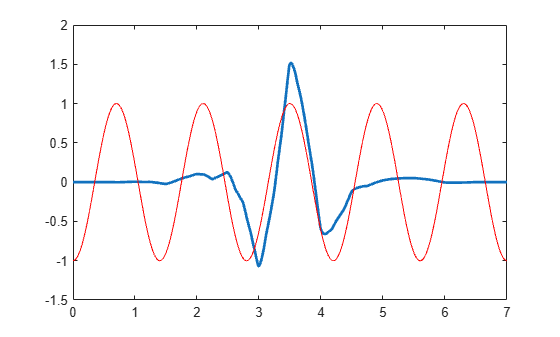Documentation

# centfrq

Wavelet center frequency

## Syntax

``FREQ = centfrq(wname)``
``FREQ = centfrq(wname,ITER)``
``[FREQ,XVAL,RECFREQ] = centfrq(wname,ITER,'plot')``

## Description

example

````FREQ = centfrq(wname)` returns the center frequency in hertz of the wavelet specified by `wname` (see `wavefun` for more information).```
````FREQ = centfrq(wname,ITER)` uses `ITER` many iterations to generate the wavelet.```
````[FREQ,XVAL,RECFREQ] = centfrq(wname,ITER,'plot')` returns the associated center frequency-based approximation `RECFREQ` on the 2ITER points grid `XVAL` and plots the wavelet function and `RECFREQ`.```

## Examples

collapse all

This example shows how to determine the center frequency in hertz for Daubechies' least-asymmetric wavelet with 4 vanishing moments.

`cfreq = centfrq('sym4');`

Obtain the wavelet and create a sine wave with a frequency equal to the center frequency, `cfreq`, of the wavelet. Use a starting phase of $-\pi$ for the sine wave to visualize how the oscillation in the sine wave matches the oscillation in the wavelet.

```[~,psi,xval] = wavefun('sym4'); y = cos(2*pi*cfreq*xval-pi); plot(xval,psi,'linewidth',2); hold on; plot(xval,y,'r');```This example shows to convert scales to frequencies for the Morlet wavelet. There is an approximate inverse relationship between scale and frequency. Specifically, scale is inversely proportional to frequency with the constant of proportionality being the center frequency of the wavelet.

Construct a vector of scales with 32 voices per octave over 5 octaves for data sampled at 1 kHz.

```Fs = 1000; numvoices = 32; a0 = 2^(1/numvoices); numoctaves = 5; scales = a0.^(0:numvoices*numoctaves-1).*1/Fs;```

Convert the scales to approximate frequencies in hertz for the Morlet wavelet.

`Frq = centfrq('morl')./scales;`

You can also use `scal2frq` to convert scales to approximate frequencies in hertz.

## Input Arguments

collapse all

Wavelet, specified as a character vector or string scalar. See `wavefun` for more information.

Number of iterations, specified by a positive integer, used to generate the wavelet `wname`. Internally, `centfrq` uses `wavefun` to generate the wavelet.

## Output Arguments

collapse all

Wavelet center frequency in hertz, returned as a scalar.

Grid points where the center frequency-based approximation to the wavelet is evaluated, returned as a real-valued vector.

Center frequency-based approximation to the wavelet, returned as a vector. Depending on the wavelet, `RECFREQ` is either a real- or complex-valued vector.